# Karta tutorial¶

## Introduction¶

Karta provides a set of tools for analysing geographical data. The organization of Karta is around a set of classes for representing vector and raster data. These classes contain built-in methods for common tasks, and are easily extended for more specialized processing. This tutorial provides a brief introduction to the elements of Karta.

Should you come across any mistakes, please file a bug or submit a pull request on Github!

The following examples are shown using Python 3, however Karta is supported on Python 2.7+ and Python 3.4+.

## Definitions¶

Vector data are data that can be treated as a set of connected or disconnected vertices. Examples might be road networks, a set of borders, geophysical survey lines, or the path taken by a bottle floating in an ocean current. In Karta, these data are classified as belonging to Point, Line and Polygon classes, and their Multipart equivalents Multipoint, Multiline, and Multipolygon. Some questions that might be asked of vector data include

• which of these points are contained in this Polygon?
• do these Lines intersect, and where?
• what is the average distance travelled by a particle?
• what municipalities does this river flow through?

Raster data is data that are typically thought of in terms of pixels or a grid of values covering a surface. Examples might be an elevation model, a satellite image, or an upstream area map. Depending on what the data represents, one might

• compute slope, aspect, and hillshades on an elevation model
• resample or interpolate a grid
• mask a land cover map according to management boundaries
• apply a pansharpening algorithm to multispectral satellite imagery
• extract an elevation profile along a path

The term coordinate reference system refers to a system of relating numerical coordinates to actual positions on Earth. Karta includes methods for geodetic calculations and basic support of projected and geographical coordinates, as well as coordinate system classes backed by pyproj.

## Vector data¶

This section demonstrates the creation and manipulation of vector data.

In :
from karta import Point, Line, Polygon, Multipoint, Multiline, Multipolygon


The Point, Line, and Polygon classes can all be instantiated by providing vertices, and optionally, associated properties. The Multipart Multipoint, Multiline, and Multipolygon classes are similar, and can additionally include part-specific tabular metadata.

In :
pt = Point((-123.1, 49.25))
print(pt)

Point(-123.1, 49.25)

In :
mpt = Multipoint([(-122.93, 48.62),
(-123.10, 48.54),
(-122.90, 48.49),
(-122.81, 48.56)],
data={"color": ["red", "blue", "green", "yellow"],
"value": [2, 1, 3, 5]})
print(mpt)
print(mpt.data)

<karta.vector.geometry.Multipoint object at 0x7fe2e041e2e8>
D[color, value]

In :
line = Line([(-124.35713, 49.31437),
(-124.37857, 49.31720),
(-124.39442, 49.31833),
(-124.40311, 49.31942),
(-124.41052, 49.32203),
(-124.41681, 49.32477),
(-124.42278, 49.32588)])
print(line)

<karta.vector.geometry.Line object at 0x7fe2e041e0f0>

In :
poly = Polygon([(-25.41, 67.03),
(-24.83, 62.92),
(-12.76, 63.15),
(-11.44, 66.82)])
print(poly)

<karta.vector.geometry.Polygon object at 0x7fe324633be0>


Each geometrical object now contains a vertex/vertices in a cartesian plane.

We may be interested in determining whether the Point created about is within the Polygon:

In :
print(poly.contains(pt))       # False

False

In :
# but this one is:
pt2 = Point((-25, 65))
print(poly.contains(pt2))      # True

True


We also can test whether the Line from above crosses the Polygon:

In :
print(line.intersects(poly))   # False

False


Or compute the shortest distance between the Point and the Line:

In :
print(line.shortest_distance_to(pt))

1.2587769197915923


There are methods for computing the nearest vertex to an external point, or the nearest point on an edge to an external point:

In :
pt = Point((0.0, 60.0))
print(poly.nearest_vertex_to(pt))
print(poly.nearest_on_boundary(pt))

2
Point(-12.301580009598124, 64.42454648846582)


The positions of objects with multiple vertices can be sliced and iterated through:

In :
subline = line[2:-2]
print(subline)

for pt in subline:
print(pt)

<karta.vector.geometry.Line object at 0x7fe2e0419da0>
Point(-124.39442, 49.31833)
Point(-124.40311, 49.31942)
Point(-124.41052, 49.32203)


A slice that takes part of a polygon returns a line.

In :
print(poly[:2])

<karta.vector.geometry.Line object at 0x7fe2e0419ac8>


Points have a distance() method that calculates the distance to another point.

In :
pt = Point((-123.1, 49.25))
pt2 = Point((-70.66, 41.52))
print(pt.distance(pt2))

53.00666467530286


By default, geometries in Karta use a planar cartesian coordinate system. If our positions are meant to be geographical coordinates, then we can provide the crs argument to each geometry at creation, as in

In :
from karta.crs import LonLatWGS84

pt = Point((-123.1, 49.25), crs=LonLatWGS84)
pt2 = Point((-70.66, 41.52), crs=LonLatWGS84)
pt.distance(pt2)

Out:
4109559.5877279835

which now gives the great circle distance between point on the Earth, in meters. We can mix coordinate systems to some degree, with Karta performing the necessary transformations in the background:

In :
from karta.crs import WebMercator

pt_web = Point((-14000000, 6300000), crs=WebMercator)
print(pt.distance(pt_web))   # distance in coordinate system units of *pt*

194483.5977009545


When the coordinate system is specified, all geometrical methods obey that coordinate system. We can use this to perform queries, such which American state capitols are within 2000 km of Mexico City?

In :
from karta.examples import us_capitols
mexico_city = Point((-99.13, 19.43), crs=LonLatWGS84)

# Filter those within 2000 km of Mexico City
nearby = list(filter(lambda pt: pt.distance(mexico_city) < 2000e3, us_capitols))
for capitol in nearby:
print("{0:4.0f} km      {1}".format(mexico_city.distance(capitol)/1e3, capitol.properties["n"]))

1785 km      Oklahoma City, Oklahoma, United States
1923 km      Montgomery, Alabama, United States
1822 km      Little Rock, Arkansas, United States
1933 km      Tallahassee, Florida, United States
1463 km      Baton Rouge, Louisiana, United States
1683 km      Jackson, Mississippi, United States
1922 km      Santa Fe, New Mexico, United States
1213 km      Austin, Texas, United States

In :
# Or, list capitols from nearest to furthest from Mexico City
distances = map(lambda pt: mexico_city.distance(pt), us_capitols)
distances_capitols = sorted(zip(distances, us_capitols))
for d, pt in distances_capitols:
print("{km:.0f} km      {name}".format(km=d/1e3, name=pt.properties["n"]))

1213 km      Austin, Texas, United States
1463 km      Baton Rouge, Louisiana, United States
1683 km      Jackson, Mississippi, United States
1785 km      Oklahoma City, Oklahoma, United States
1822 km      Little Rock, Arkansas, United States
1922 km      Santa Fe, New Mexico, United States
1923 km      Montgomery, Alabama, United States
1933 km      Tallahassee, Florida, United States
2027 km      Phoenix, Arizona, United States
2155 km      Atlanta, Georgia, United States
2199 km      Topeka, Kansas, United States
2214 km      Nashville, Tennessee, United States
2225 km      Jefferson City, Missouri, United States
2320 km      Denver, Colorado, United States
2382 km      Lincoln, Nebraska, United States
2414 km      Columbia, South Carolina, United States
2429 km      Springfield, Illinois, United States
2467 km      Cheyenne, Wyoming, United States
2495 km      Frankfort, Kentucky, United States
2510 km      Des Moines, Iowa, United States
2576 km      Indianapolis, Indiana, United States
2661 km      Salt Lake City, Utah, United States
2694 km      Charleston, West Virginia, United States
2708 km      Raleigh, North Carolina, United States
2752 km      Columbus, Ohio, United States
2769 km      Pierre, South Dakota, United States
2777 km      Madison, Wisconsin, United States
2885 km      Saint Paul, Minnesota, United States
2904 km      Richmond, Virginia, United States
2922 km      Lansing, Michigan, United States
2947 km      Carson City, Nevada, United States
3025 km      Sacramento, California, United States
3030 km      Washington, District of Columbia, United States
3041 km      Bismarck, North Dakota, United States
3070 km      Annapolis, Maryland, United States
3118 km      Boise, Idaho, United States
3137 km      Harrisburg, Pennsylvania, United States
3150 km      Dover, Delaware, United States
3235 km      Helena, Montana, United States
3274 km      Trenton, New Jersey, United States
3506 km      Albany, New York, United States
3517 km      Hartford, Connecticut, United States
3585 km      Salem, Oregon, United States
3605 km      Providence, Rhode Island, United States
3665 km      Boston, Massachusetts, United States
3688 km      Concord, New Hampshire, United States
3696 km      Montpelier, Vermont, United States
3748 km      Olympia, Washington, United States
3879 km      Augusta, Maine, United States
5172 km      Juneau, Alaska, United States
6093 km      Honolulu, Hawaii, United States


All of the above calculations are performed on a geoid. The LonLatWGS84 coordinate system means to use geographical (longitude and latitude) coordinates on the WGS 84 ellipsoid.

### Associated data¶

By using the data keyword argument, additional data can be associated with a Multipart vector geometry. The data can be a list or a dictionary of lists.

In :
mp = Multipoint([(1, 1), (3, 1), (4, 3), (2, 2)],
data={"species": ["T. officianale", "C. tectorum",
"M. alba", "V. cracca"]})


These data live in the .data attribute, which is a Table instance. For convenience, the data can also be accessed via the .d attribute, which provides a streamlined syntax supporting key-lookups, indexing, and slicing.

In :
mp.d

Out:
Indexer(D[species])
In :
mp.d["species"]

Out:
['T. officianale', 'C. tectorum', 'M. alba', 'V. cracca']
In :
mp.d[1:3]

Out:
{'species': ['C. tectorum', 'M. alba']}

The data are propagated through indexing operations on their parent geometry:

In :
pt = mp
print(pt, "-", pt.properties["species"])

Point(4.0, 3.0) - M. alba


Geometry-level metadata at the geometry level can be provided using the properties keyword argument, which accepts a dictionary. Derived geometries carry the properties of their parent geometry.

In :
poly = Polygon([(-25.41, 67.03),
(-24.83, 62.92),
(-12.76, 63.15),
(-11.44, 66.82)],
properties={"geology": "volcanic",
"alcohol": "brennivin"})
print(poly[0:3].properties)

{'geology': 'volcanic', 'alcohol': 'brennivin'}


### Visualizing and importing/exporting data¶

The get_coordinate_lists method and coordinates attribute provide lists of coordinates for plotting or data export.

Higher-level plotting operations are provided by the separate karta.plotting submodule, not described here.

In :
import matplotlib.pyplot as plt
%matplotlib inline
plt.plot(*line.coords())

Out:
[<matplotlib.lines.Line2D at 0x7fe2dbc026a0>]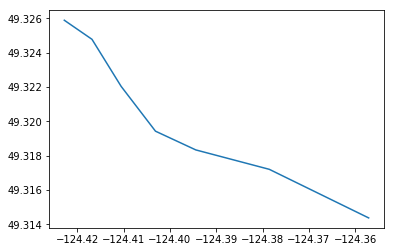Data can be read from and written to several common formats, including ESRI shapefiles (through bindings to the pyshp module), GeoJSON, and GPX. Convenience functions are kept in the karta.vector.read namespace.

Each geometry has appropriate methods to write data:

In :
line.to_shapefile("line.shp")
pt_web.to_geojson("point.geojson")


## Raster data¶

Raster data are primarily represented by the karta.RegularGrid class. RegularGrid instances have a CRS, a Null-data value, a geotransform, and one or more bands, which containing the actual data.

### Bands¶

To provide flexibility, different band classes are provided by karta.raster.bands using different strategies for data storage.

• The simplest case, SimpleBand, uses a numpy array to store all data. This makes it reasonably fast, but can be memory-hungry with large rasters.
• The default case, CompressedBand, uses chunking and compression via the blosc library to reduce the memory footprint of the raster data at a small speed cost.
• GdalFileBand reads data directly from a valid GDAL datasource, using the least memory but performing the slowest.

Note: GdalFileBand doesn't currently handle all raster operations supported by the other band types. Many operations implicitly convert to in-memory CompressedBand representation.

In :
import numpy as np
from karta.raster import RegularGrid, SimpleBand, CompressedBand, read_gtiff

print(ls8.bands)     # list of one CompressedBand instance

[<karta.raster.band.CompressedBand object at 0x7fe2dbbcd400>]

In :
# Print grid dimensions
print(ls8.size)

(15861, 15601)

In :
# Print grid extent
print(ls8.extent())

(319200.0, 553200.0, 4503900.0, 4741800.0)

In :
# Visualize data
plt.imshow(ls8[::10,::10, 0], origin="bottom", extent=ls8.extent(), cmap=plt.cm.binary, vmin=3e3, vmax=10e3)
plt.colorbar()

Out:
<matplotlib.colorbar.Colorbar at 0x7fe2dbb6a198>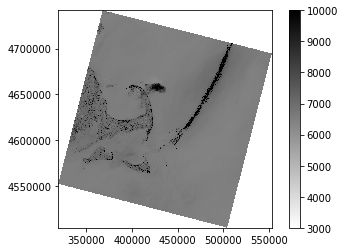When opening or creating a RegularGrid, a non-default band type can be specified as a keyword argument. The following code re-opens the same grid as a SimpleBand and verifies that all data are the same.

In :
ls8_numpy = read_gtiff("LC08_L1TP_011031_20180930_20181010_01_T1_B8.TIF", bandclass=SimpleBand)
np.all(ls8[:,:] == ls8_numpy[:,:])    # True

Out:
True

In the above, the slice syntax [:,:] is used to get an array of all grid data. Because the grid ls8 has only a single band in this case, the data array has two dimensions. The normal simple slicing rules apply, i.e. one can do things like:

In :
subgrid = ls8[2000:3000, 4000:4500]
print(subgrid.shape)

(1000, 500, 1)

In :
every_other = ls8[::2, ::2]
print(every_other.shape)

(7931, 7801, 1)

In :
ls8.transform

Out:
(319192.5, 4503892.5, 15.0, 15.0, 0.0, -0.0)

Grid geolocation is based on an affine matrix transformation represented by the .transform attribute, as well as an associated coordinate system under the .crs attribute. The extent and bounding box of the grid can be retrieved using the respective properties:

In :
print(ls8.bbox())
print(ls8.extent())

(319192.5, 4503892.5, 553207.5, 4741807.5)
(319200.0, 553200.0, 4503900.0, 4741800.0)


The extent is of the form (xmin, xmax, ymin, xmax), and refers to grid centers. The bbox is of the form (xmin, ymin, xmax, ymax), and refers to grid edges.

To get swaths of grid coordinates conveniently and in arbitrary coordinate systems, used the .coordinates() method, which returns a CoordinateGenerator instance that can be indexed to generate coordinate pairs.

In :
coords = ls8.coordinates(crs=LonLatWGS84)
print(coords[0,0])
print(coords[-1,-1])
print(coords[:5,:5])

(-71.13895087509408, 40.66617380088692)
(-71.13912389811426, 40.66603547707743)
(array([[-71.13895088, -71.13877354, -71.1385962 , -71.13841886,
-71.13824152],
[-71.13895519, -71.13877785, -71.13860051, -71.13842317,
-71.13824583],
[-71.13895951, -71.13878217, -71.13860483, -71.13842749,
-71.13825015],
[-71.13896382, -71.13878648, -71.13860914, -71.1384318 ,
-71.13825446],
[-71.13896814, -71.1387908 , -71.13861346, -71.13843612,
-71.13825878]]), array([[40.6661738 , 40.66617709, 40.66618037, 40.66618366, 40.66618695],
[40.66630884, 40.66631212, 40.66631541, 40.6663187 , 40.66632198],
[40.66644388, 40.66644716, 40.66645045, 40.66645373, 40.66645702],
[40.66657891, 40.6665822 , 40.66658548, 40.66658877, 40.66659206],
[40.66671395, 40.66671724, 40.66672052, 40.66672381, 40.66672709]]))


### Grid sampling¶

Nearest-neighbour and bilinear sampling of grid points is supported via the .sample() method. It is also possible to resample the full grid at a new resolution, or to sample along a profile.

In :
val = ls8.sample(Point((500000, 8750000), crs=ls8.crs))     # sample a point using the grid CRS
print(val)



In :
# Resample grid at 100 m postings:
ls8_coarse = ls8.resample(100, 100)
print("Original resolution:", ls8.resolution)
print("Resampled resolution:", ls8_coarse.resolution)

Original resolution: (15.0, 15.0)
Resampled resolution: (100.0, 100.0)

In :
# Generate a line and sample at intervals along it
transit = Line(zip(np.linspace(350000, 450000), np.linspace(4550000, 4700000)), crs=ls8.crs)
mp, val = ls8.profile(transit)

plt.subplot(2, 1, 1)
x, y = mp.coords()
plt.scatter(x, y, c=val.flatten(), edgecolor="none", cmap=plt.cm.binary)

plt.subplot(2, 1, 2)
dist = Line(mp).cumulength()    # convert sample points to a line and extract
# the cumulative distance along it
plt.plot(dist, val)

Out:
[<matplotlib.lines.Line2D at 0x7fe2db936588>]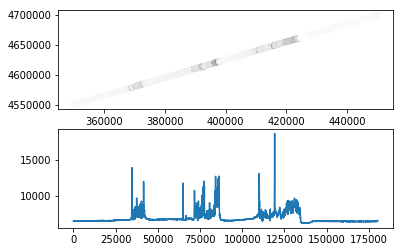### Grid resizing¶

Grids can be trimmed or expanded using the .resize() method, which takes a new bounding box as an argument.

Note

When getting raster data, the array provided by slicing is not necessarily a view of the underlying data, and may be a copy instead. Modifying the array is not guaranteed to modify the raster. When the raster data must be replaced by an element-wise computation, use the Grid.apply(func) method, which operates in-place. The apply method may be chained.

# Example
grid.apply(lambda x: x**2) \
.apply(np.sin) \
.apply(lambda x: np.where(x < 0.5, grid.nodata, x))

This handles nodata pixels automatically. If the raster data must be replaced by arbitrary data, set it explicitly with Grid[:,:] = ....

# Example
grid[:,:] = np.convolve(np.ones([3,3])/9.0, grid[:,:], mode='same')
In :
ls8_small = ls8.resize((350000, 4600000, 450000, 4650000))
plt.imshow(ls8_small[:,:,0], origin="bottom", extent=ls8_small.extent(), cmap=plt.cm.binary, vmin=3e3, vmax=12e3)

Out:
<matplotlib.image.AxesImage at 0x7fe2db8aada0>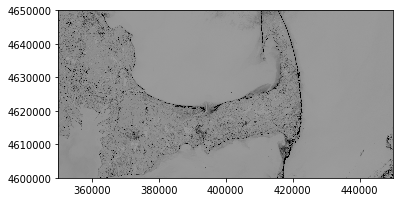### Creating RegularGrid instances¶

New RegularGrid instances are created by specifying a geotransform. The geotransform is represented by a tuple of the form

transform = (xll, yll, dx, dy, sx, sy)

where xll and yll are the coordinates of the lower left grid corner, dx and dy specify resolution, and sx and sy specify grid skew and rotation.

The following creates an empty global grid with 5 degree resolution, oriented "north-up" and "east-right", and then plots the pixel centers:

In :
from karta.crs import LonLatWGS84, GallPetersEqualArea
newgrid = RegularGrid((-180, -80, 5, 5, 0, 0), values=np.zeros((160//5, 360//5)), crs=LonLatWGS84)

# visualize the coordinate positions on a Gall-Peters projection
coords = newgrid.coordinates(crs=GallPetersEqualArea)
x, y = coords[:,:]
_ = plt.plot(x, y, ".k", ms=2)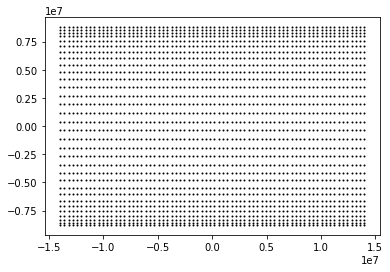TODO